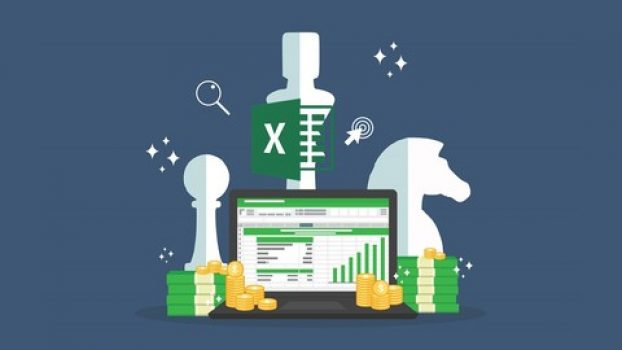# Microsoft Excel for Finance, Accounting & Financial AnalysisMicrosoft Excel for Finance, Accounting & Financial AnalysisNow, Course instructor offering 100%OFF on the original price of the course and its limited time offer. Enroll the course before the coupon expired Once you’re enrolled for the course, you can start it whenever and complete it at your own pace. it will never expire on your account.

## What you’ll learn

• Accounting functions in Microsoft Excel
• Finance functions in Microsoft Excel

Excel:

• Basic navigation and cell formatting
• Basic maths functions like plus, minus, multiply and divide

Accounting:

• Concept of depreciation and methods of depreciation

Finance:

• Concept of Time Value of Money
• Present Value and Future Value concept
• Conversion from present value to future value, and from future value to present value
• Concept of Discount factor and Annuity
• Concept and calculation of NPV
• Concept of calculation of IRR

Topics Covered:

• Calculation of depreciation with Excel formulas
• Calculation of PV and FV with Excel formulas
• Calculation of NPV with Excel formulas
• Calculation of IRR with Excel formulas

## Who this course is for:

• Accounting and Finance Students
• Accounting and Finance Professionals
• Aspiring Financial Analysts
• Business owners with finance knowledge

#### Can I download Microsoft Excel for Finance, Accounting & Financial Analysis course?